# resizing a curved polygon causes small "jogs" in the resized polygon

Hi,

I'm using the following resizing DRC script to resized curved polygons (several data points per degree of curve). The case below copies all layers from input to output, and creates (4,0) which is (2,0) resized by 3.5.

``````if \$input && \$output
source(\$input)
target(\$output)
end
deep
source.layers.each do |lp|
input(lp).output(lp)
if lp.layer == 2 && lp.datatype == 0
input(lp).sized(3.5).output(4, 0)
end
end
``````

The resizing appears to work properly, except that there are sporadic "jogs" in the polygon of the resized layer. These jogs are 1 nm long (the database unit), and are DRC violations for my process. Changing the data in the original curved polygon (fewer data points per degree of arc, for instance) just puts the "jogs" in different locations. There are no jogs in the original curve.

So my questions are...(1) is there something wrong with the way I'm doing this? and if not, (2) is there a way to write a script (that can be called at the command line) which removes the jogs...perhaps by checking to see if a polygon contains a pair of points that are closer together than, say, 2 nm, and then deleting one of them? Currently, I'm stuck removing them manually, which is not sustainable.

Thanks,
Brian

• Hi Brian,

could you provide an example for such a polygon? You can .zip a GDS file and attach it here. Code without sample data is not pretty useful.

Basically 3.5µm is a pretty large sizing. The sizing feature works edge based by shifting edges and reconnecting them. The reconnection step is well defined only for positive bias and convex polygons without acute (angle < 90 degree) angles. Even then however, it needs to snap the intersection point for non-orthogonal edges. This implies a numerical offset, but still for every original vertex the output polygon will have a one corresponding vertex.

For concave polygons or negative bias, the algorithm needs to shorten edges or even eliminate them. This is a problem specifically for large sizing values when the edge lengths are smaller compared to the sizing value. The edge elimination step is an elaborate process involving a boolean merge to remove the potentially manifold internal intersections and render the final polygon. Snapping effects leading to jogs a likely in this case.

So I assume your polygons are concave, the edges are short compared to the sizing value and non-orthogonal. In this case, you're expecting a little too much from an edge-based sizing.

To solve your problem I'd first discuss the validity of a DRC flagging small jogs. I cannot imagine and physical motivation for such a rule. My experience is that such rules are present to flag "sloppiness" of layout or act against "creative" layout such as "stairs" with small steps to emulate a 45 degree edge. Sometimes, short edges are forbidden to enable layout post-processing steps such as optical proximity correction, but a sizing value of 3.5µm hints towards a technology node where this is not in place.

As another alternative, you can try the smooth feature. It is supposed to eliminate such jogs, but it does not always do so to preserve the bounding box of a feature for example.

Matthias

• My impression is that the problem involves a convex shape,
and vertex-snapping perhaps is making local concavities that
become "notch" errors?

I guess there "could" be logic that makes all vertex snapping
"radially outward", but this probably ought to be user's
choice, with options to do "plain snap", "outie snap", "innie snap"?
Obviously you can't have an all-concave structure though,
so maybe "all innie" is worthless.

Maybe a touchup script that will locate "innies" and offer to
"snap next outward X", "snap next outward Y", "snap next
best outward" that you could use to chew on just one

Then again maybe DRC should just have enough tolerance
to accept tiny "warts" on large sized objects (but then you'd
have no negotiate waivers on any discrepancies between
local DRC rules and golden tape-in deck).

• I tried something out.

A multi-vertex polygon can have a single vertex selected by
Partial tool: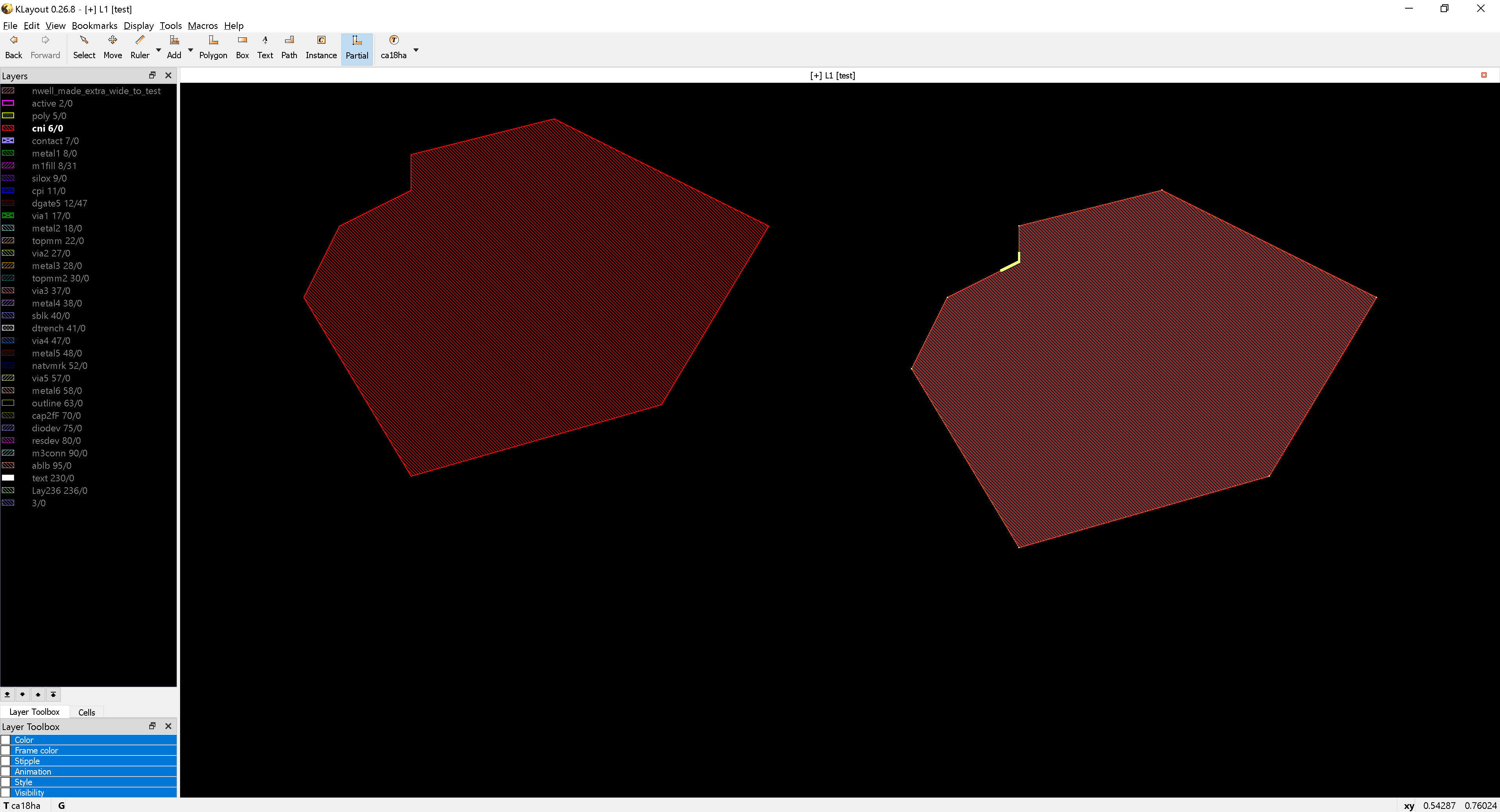There you can see an "innie" vertex selected.

Now if you delete that vertex, the shape becomes fully convex.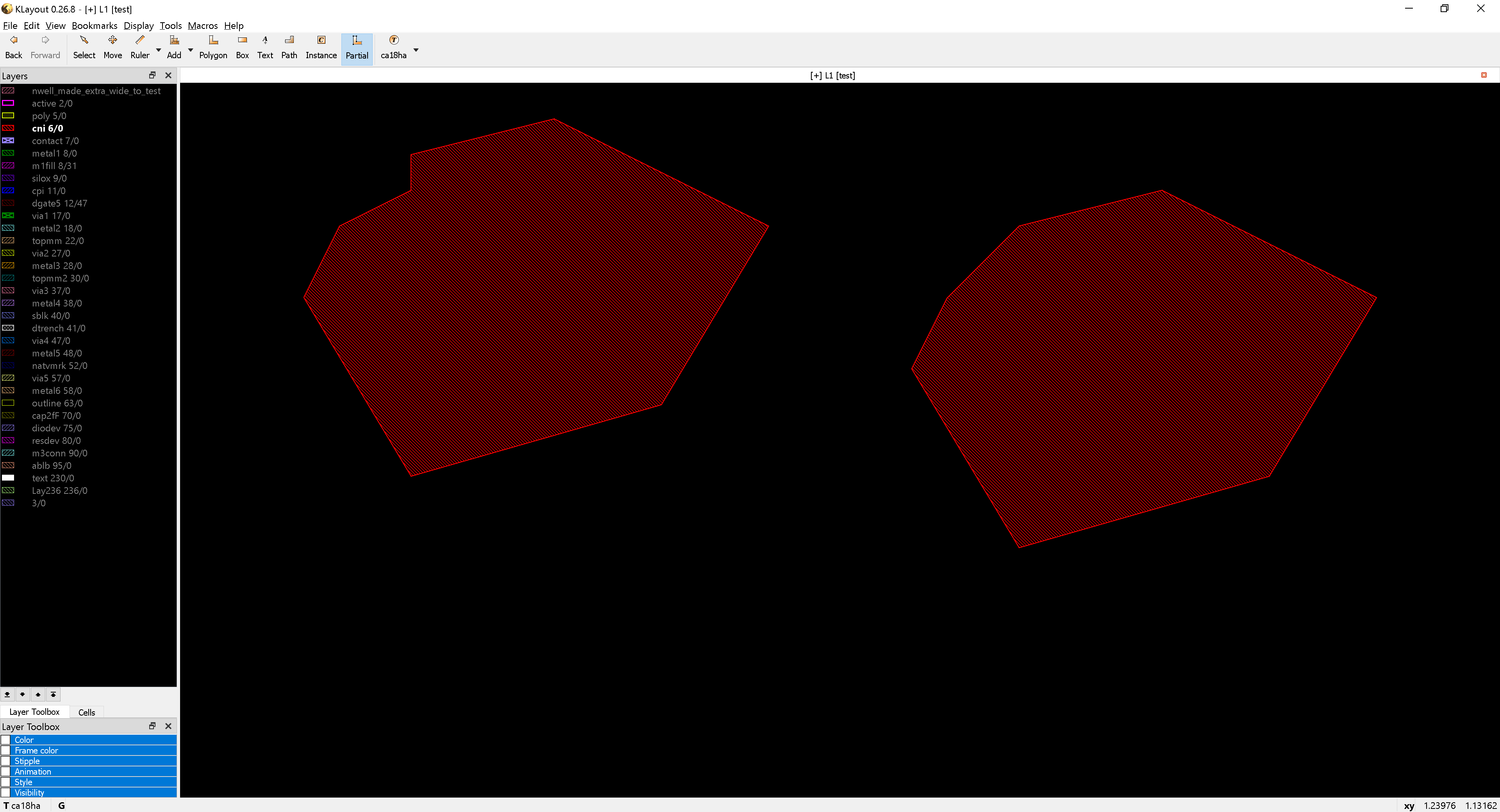So seems like, if you could identify by script a vertex (from the
property list) which is radially less-outward than its connected
neighbor vertices, that you could either delete the vertex, or
calculate the "least further out, on grid, convex-making" new
point. Either way, making sure there will be no "notch" errors
as there would be no notches.

• Thanks for your comments. This is a photonic circuit layout, so the issue, I suppose, is that the original polygon is a curve (which requires very high density of data points), whereas the resizing is to create fill keepout. And yes, the jogs occur only in concave regions.

For now, I'm manually deleting the offending data points in the resized version. I've somewhat automated it in Matlab (I double-click the polygon, cut and paste the data into a matlab script, which removes the jogs, then I cut and paste the results back into KLayout, replacing the original data. Ugly but effective).

I suppose it would be much easier in the long run to create a copy of the high-density curve on a dummy layer, but with a much lower data density, and then to resize that layer to create the fill keepout.

• The smooth function actually reduces the density. The definition of this function is to delete points if the resulting modified curve stays close to the original curve within a certain limit. I think you should try this.

I also conducted some experiment:

My input is a 2000 points circle with 1µm radius. The plain sizing

``````l = input(1, 0)
l.sized(3.5).output(100, 0)
``````

Gives a jagged circle like this: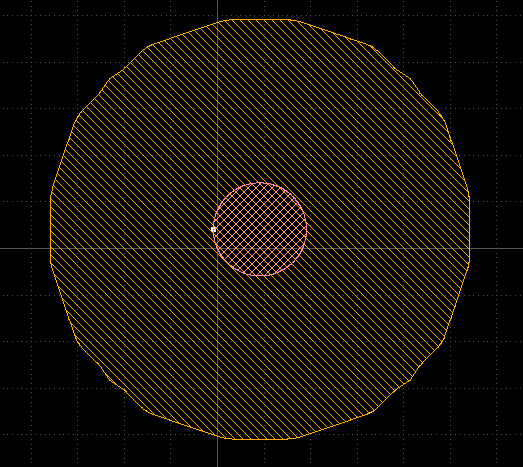I do not see jogs, but the outline is not smooth: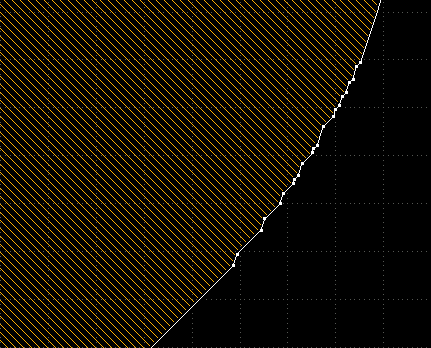If I apply smoothing before (with an accuracy of 10nm), the result is much better:

``````l = input(1, 0)
l.smoothed(0.01).sized(3.5).output(101, 0)
``````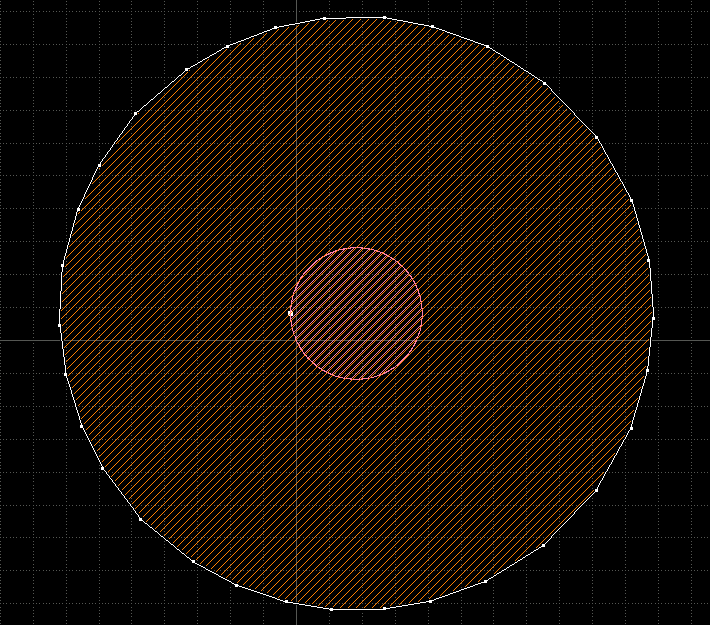Matthias